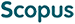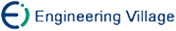姚宏亮, 王秀芳, 胡大伟, 王浩, 茆美琴. 多Agent动态影响图的一种混合近似推理算法[J]. 计算机研究与发展, 2011, 48(4): 584-591.
 引用本文: 姚宏亮, 王秀芳, 胡大伟, 王浩, 茆美琴. 多Agent动态影响图的一种混合近似推理算法[J]. 计算机研究与发展, 2011, 48(4): 584-591.Yao Hongliang, Wang Xiufang, Hu Dawei, Wang Hao, Mao Meiqin. A Hybrid Approximate Inference Algorithm for Multi-Agent Dynamic Influence Diagrams[J]. Journal of Computer Research and Development, 2011, 48(4): 584-591.
 Citation: Yao Hongliang, Wang Xiufang, Hu Dawei, Wang Hao, Mao Meiqin. A Hybrid Approximate Inference Algorithm for Multi-Agent Dynamic Influence Diagrams[J]. Journal of Computer Research and Development, 2011, 48(4): 584-591.## A Hybrid Approximate Inference Algorithm for Multi-Agent Dynamic Influence Diagrams

Abstract: Multi-agent dynamic influence diagrams (MADIDs) are fit for modeling multi-agent systems in dynamic environment, where structural relations among agents are represented in the local factorial probability form. A major problem of probability graphical models inference is that it is difficult to realize tradeoff between accuracy and complexity computational of approximate inference. In general, the approximation inference methods can improve computational efficiency, but it will also induce the loss of accuracy. BK algorithm and particle filter are two important approximate algorithms for dynamic probability models. BK algorithm has considerable computational efficiency, but induces the error of the inference. PF algorithm can approximate arbitrary distribution, but with the high dimension problem of computation. Hybrid approximate inference(HAI) algorithm for multi-agent dynamic influence diagrams (MADIDs) is presented by combining the advantage of BK and particle filter, and HAI algorithm converts the distribution of MADIDs into the local factorial form. Based on decomposability of probability graph models, HAI algorithm decomposes MADIDs to prototype junction tree, and particle filter is executed on these clusters with lesser scale complexity to achieve local optimal estimation, while BK for other clusters; in addition, separator clusters are induced for decreasing inference errors in HAI algorithm. Simulation results show that the proposed algorithm improves computational precision notably comparied with BK algorithm and PF algorithm, and the algorithm is an efficient approximation inference method of MADIDs and can establish a tradeoff between accuracy and complexity of approximate distribution./下载:  全尺寸图片 幻灯片
• 分享
• 用微信扫码二维码

分享至好友和朋友圈